## 机器学习：主成分分析原理及应用

6670 字 · 220 阅读 · 2023 年 05 月 15 日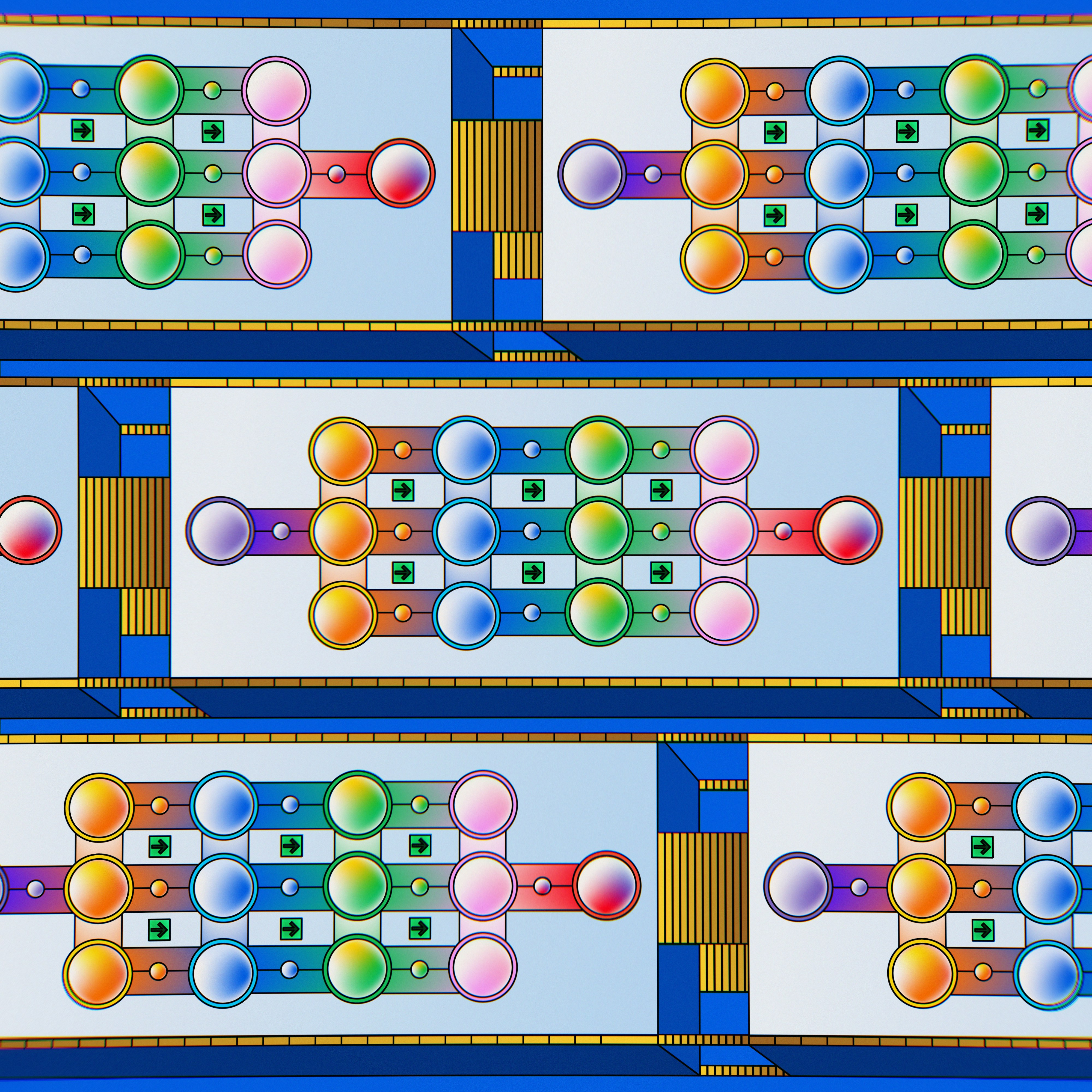• 向量的基
• 向量映射
• 方差和协方差
• 特征值和特征向量
• 主成分分析计算

### 向量的基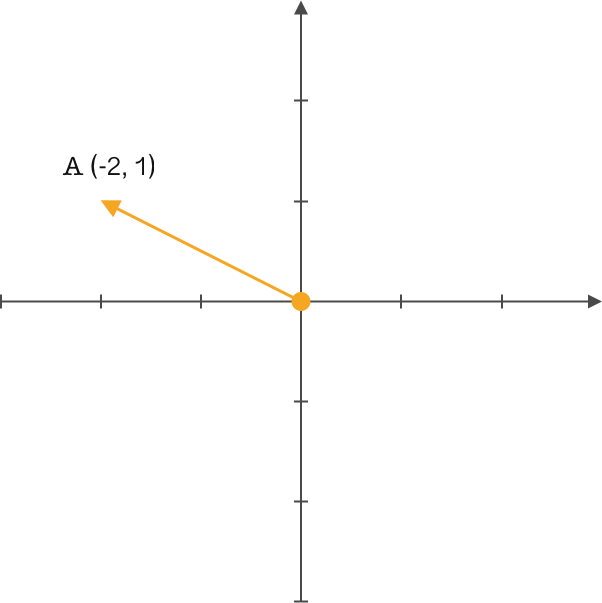$A(-2,1)$ 这应该是大家想到的结果。没错，在高中时期我们通常都会这样定义。但线性代数中就会有另一个概念：基。

### 重新定义向量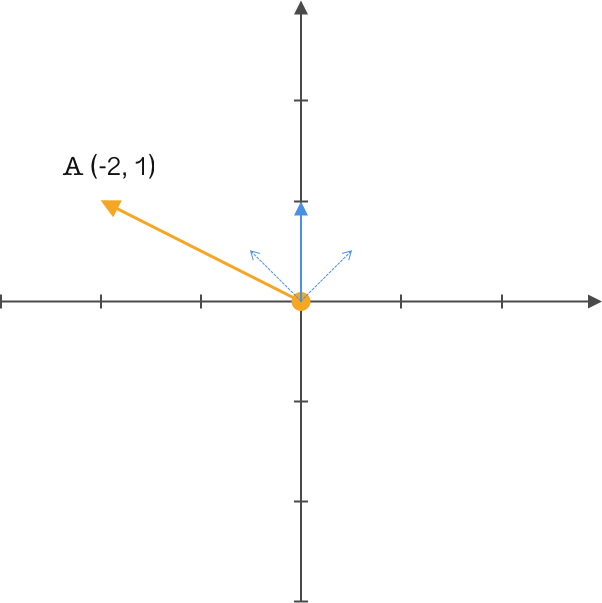$$\left( \begin{array}{cc}{\frac{\sqrt{2}}{2}} & {\frac{\sqrt{2}}{2}} \\ {-\frac{\sqrt{2}}{2}} & {\frac{\sqrt{2}}{2}}\end{array}\right) \left( \begin{array}{c}{-2} \\ {1}\end{array}\right)=\left( \begin{array}{c}{-\frac{\sqrt{2}}{2}} \\ {\frac{3 \sqrt{2}}{2}}\end{array}\right)$$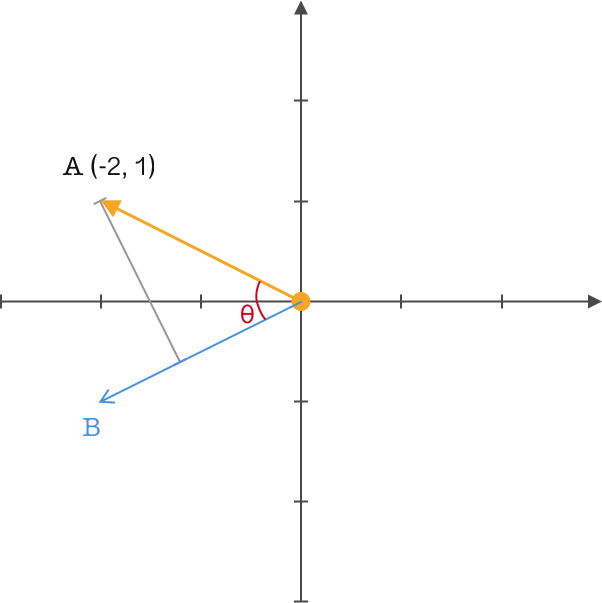$$A\cdot B=\left | A \right |\left | B \right |cos(\theta )$$

$$A\cdot B=\left | A \right |cos(\theta )$$

### 重新定义矩阵

$$\left( \begin{array}{cc}{\frac{\sqrt{2}}{2}} & {\frac{\sqrt{2}}{2}} \\ {-\frac{\sqrt{2}}{2}} & {\frac{\sqrt{2}}{2}}\end{array}\right) \left( \begin{array}{cccc}{-2} & {2} & {-2} & {2} \\ {1} & {1} & {-1} & {-1}\end{array}\right)=\left( \begin{array}{cccc}{\frac{-\sqrt{2}}{2}} & {\frac{3 \sqrt{2}}{2}} & {\frac{-3 \sqrt{2}}{2}} & {\frac{\sqrt{2}}{2}} \\ {\frac{3 \sqrt{2}}{2}} & {\frac{-\sqrt{2}}{2}} & {\frac{\sqrt{2}}{2}} & {\frac{-3 \sqrt{2}}{2}}\end{array}\right)$$

$$\left( \begin{array}{cc}{\frac{\sqrt{2}}{2}} & {\frac{\sqrt{2}}{2}} \\ {\frac{-\sqrt{2}}{2}} & {\frac{\sqrt{2}}{2}} \\ {\frac{\sqrt{2}}{2}} & {\frac{-\sqrt{2}}{2}}\end{array}\right) \left( \begin{array}{cc}{-2} & {2} \\ {1} & {1}\end{array}\right)=\left( \begin{array}{cc}{\frac{-\sqrt{2}}{2}} & {\frac{3 \sqrt{2}}{2}} \\ {\frac{3 \sqrt{2}}{2}} & {\frac{-\sqrt{2}}{2}} \\ {\frac{-3 \sqrt{2}}{2}} & {\frac{\sqrt{2}}{2}}\end{array}\right)$$

$$\left( \begin{array}{c}{M_{1}} \\ {M_{2}} \\ {\vdots} \\ {M_{i}}\end{array}\right) \left( \begin{array}{cccc}{N_{1}} & {N_{2}} & {\dots} & {N_{j}}\end{array}\right)=\left( \begin{array}{cccc}{M_{1} N_{1}} & {M_{1} N_{2}} & {\cdots} & {M_{1} N_{j}} \\ {M_{2} N_{1}} & {M_{2} N_{2}} & {\cdots} & {M_{2} N_{j}} \\ {\vdots} & {\vdots} & {\ddots} & {\vdots} \\ {M_{i} N_{1}} & {M_{i} N_{2}} & {\cdots} & {M_{i} N_{j}}\end{array}\right)$$

### 主成分分析 PCA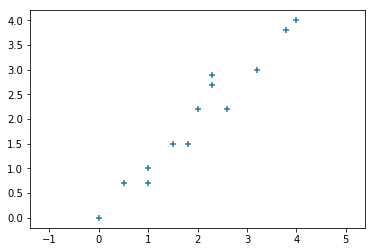import numpy as np
import matplotlib.pyplot as plt
%matplotlib inline

x = np.array([0, 0.5, 1, 1, 1.5, 1.8, 2, 2.3, 2.3, 2.6, 3.2, 3.8, 4])
y = np.array([0, 0.7, 1, 0.7, 1.5, 1.5, 2.2, 2.9, 2.7, 2.2, 3, 3.8, 4])

arr = np.array([x, y])
plt.axis("equal")
plt.scatter(x, y, marker='+')


base = np.array([np.sqrt(2)/2, np.sqrt(2)/2])  # 基向量
base


result_eye = np.dot(base, arr)
result_eye


### 方差和协方差

$$var(x) = \frac{1}{n}\sum_{i=1}^{n}(X_{i}-\bar{X})(X_{i}-\bar{X})$$

$$cov(x) = \frac{1}{n}\sum_{i=1}^{n}(X_{i}-\bar{X})(Y_{i}-\bar{Y})$$

### 协方差矩阵

$$C(x_{a},x_{b})=\frac{1}{n}\sum_{i=1}^{n}(x_{ai}-\bar{x}_{a})(x_{bi}-\bar{x}_{b})$$

$$C \left( \begin{array}{ccc}{\operatorname{cov}(x, x)} & {\operatorname{cov}(x, y)} & {\operatorname{cov}(x, z)} \\ {\operatorname{cov}(y, x)} & {\operatorname{cov}(y, y)} & {\operatorname{cov}(y, z)} \\ {\operatorname{cov}(z, x)} & {\operatorname{cov}(z, y)} & {\operatorname{cov}(z, z)}\end{array}\right)$$

### 特征值和特征向量

$$Av = \lambda v$$

covMat = np.cov(arr)  # 计算协方差矩阵
covMat


eigVal, eigVec = np.linalg.eig(np.mat(covMat))  # 获得特征值、特征向量
eigVal, eigVec


eigValInd = np.argsort(-eigVal)  # 特征值降序排序
bestVec = eigVec[:, eigValInd[:1]]  # 取最好的一个特征向量
bestVec


result_cov = np.dot(arr.T, bestVec)
result_cov


fig, axes = plt.subplots(1, 2, figsize=(10, 5))
axes.scatter(result_cov.flatten().A, [
0 for i in range(len(x))], marker='+')
axes.scatter(result_eye, [0 for i in range(len(x))], marker='+')


np.var(result_cov), np.var(result_eye)


### 高维数据降维处理

$590$ 个特征不算太多，但也是一个很大的维度了。所以，这里使用降维的方式处理数据集，得到一个维度更低，但仍然保留绝大部分的原始信息的新数据集。

import pandas as pd

df.describe()


data = df.values
mean = np.mean(data, axis=0)
data = data - mean
data


covMat = np.cov(data, rowvar=False)
covMat


eigVal, eigVec = np.linalg.eig(np.mat(covMat))
plt.plot(eigVal[:30], marker='o')


sum(eigVal[:4]), sum(eigVal)*0.9


eigValInd = np.argsort(-eigVal)  # 特征值降序排序
bestVec = eigVec[:, eigValInd[:4]]  # 取最好的 4 个特征向量
bestVec

np.dot(data, bestVec)


from sklearn.decomposition import PCA

pca = PCA(n_components=4)
pca.fit_transform(data)


### 系列文章•